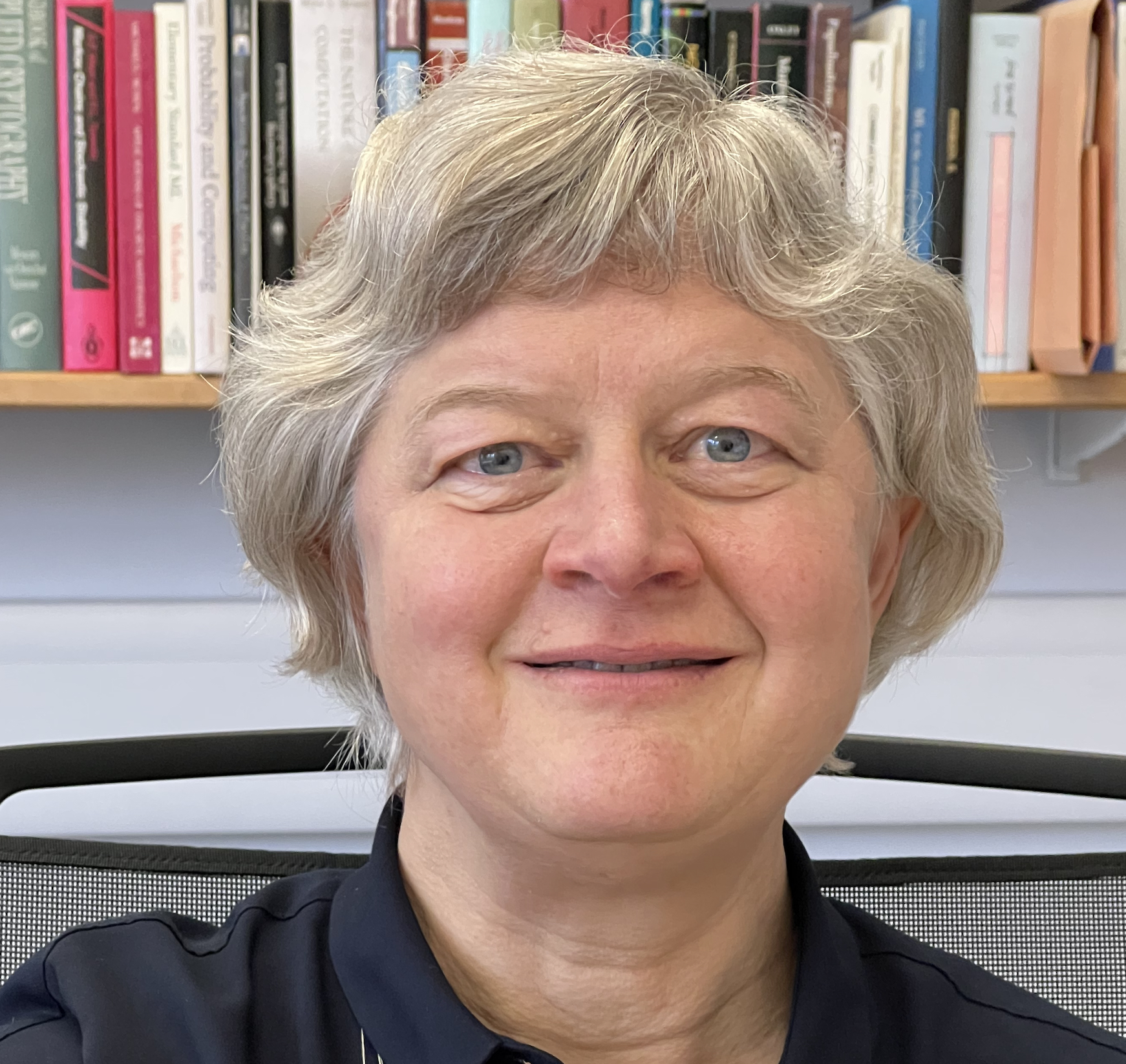• Professor Leslie Ann Goldberg

• Email (CS dept or University of Oxford): head-of-dept@cs.ox.ac.uk
• Email (my research, my teaching, or St Edmund Hall): leslie.goldberg@seh.ox.ac.uk
• Office: 256 Wolfson Building

• PA: Jayne Bullock Jayne.Bullock@cs.ox.ac.uk +44 1865 283503

• Address: Department of Computer Science, University of Oxford,
Wolfson Bldg, Parks Rd,
Oxford OX1 3QD United Kingdom

I am the Head of the Department of Computer Science (and a Professor of Computer Science) at the University of Oxford. I am also a Senior Research Fellow at St Edmund Hall. I co-teach the MSc and Part C course Probability and Computing with Marc Roth.

Faculty hiring in our Department: See here.

Prospective PhD students: Our deadlines have passed for entry in Autumn of 2023. I will be looking for new DPhil (PhD) students to join me in Autumn of 2024. For information about how to apply and deadlines, see https://www.ox.ac.uk/admissions/graduate/courses/dphil-computer-science. Prospective students should feel free to get in touch if any of the projects described here sound interesting, or if you have other related ideas in algorithms or complexity theory.

## Research Interests

I am interested in foundational questions in Algorithms and Complexity Theory. A primary goal in this area is to figure out which computational problems can be solved with fast algorithms and to discover fast algorithms for solving these problems. The other primary goal is to figure out which problems provably can't be solved with fast algorithms, and to prove that no fast algorithms exist for solving these problems (usually relying on conjectures from the field of complexity theory).

I am especially interested in randomised algorithms, which are algorithms that use probabilistic methods to solve problems. Randomised algorithms arise in a huge variety of computational applications including algorithms for communication and information spread in networks, algorithms for machine learning, and algorithms for analysing computational models from statistical physics. I am particularly interested in the rigorous, mathematical analysis of these algorithms - proving results about how long the algorithms take, and how accurate they are.

My current research projects include
• Contention resolution. A backoff protocol is a simple and elegant randomised algorithm for communicating in a shared channel called a Multiple Access Channel. An example is the Ethernet protocol. Even though Ethernet-like protocols are used everywhere, it turns out that the most fundamental mathematical questions about backoff protocols are still open. Aldous conjectured in 1987 that, in the most pure setting, for every positive arrival rate, every backoff protocol is unstable (which informally means that unsent messages build up more and more as time goes on). Together with John Lapinskas from Bristol, I am working on this conjecture and related questions. Our most recent paper, making progress on the conjecture, is here.

• Randomised algorithms for sampling and approximate counting. Work in this area focusses on two related topics: algorithms for random sampling and randomised algorithms for approximate counting. These two topics are closely related and the same basic techniques apply to both, in particular rigorous analysis of Markov Chain Monte Carlo algorithms (see for example this paper).

A lot of my current work in this area is joint with Andreas Galanis at Oxford. Here is a recent paper (with Galanis, Guo, and Herrera-Poyatos) about algorithms for sampling satisfying assignments of Boolean formulas. Most of the maths that gets used in analysing algorithms for random sampling and approximate counting comes from probability or combinatorics, but sometimes other things can come up. Here is a recent paper (with Bezakova, Galanis and Stefankovic) where the analysis of approximate counting algorithms relied more on complex dynamics (which turned out to be fun!) Andreas and I are currently working on sampling algorithms for spin systems with our jointly-supervised DPhil student, Paulina Smolarova.

• The study of counting complexity (as part of complexity theory) goes back to Valiant in the 1970s, but in the context of randomness and approximation there are many additional challenges. Here is an early paper with Dyer, Greenhill, and Jerrum, which identifies a key complexity class for approximate counting, centred around a problem called #BIS, which is the problem of approximately counting the independent sets of a bipartite graph. By now there are many computational problems and classes of computational problems which are known to be equivalent to #BIS, but we still don't know whether it even has a fast approximation algorithm (with or without randomness), and this is a tantalising open problem.

• Analysing processes on networks. The Moran process is a process which models the spread of genetic mutations in graphs. The main idea is to derive rigorous probabilistic bounds on how these mutations spread over time (as a function of the graph). I am currently working on these questions with Marc Roth and John Lapinskas. Some of my work in the area includes this paper (joint with Galanis, Goebel, Lapinskas and Richerby) and this paper (joint with Lapinskas and Richerby). There are other interesting related models. For example, the voter model, which models the spread of competing ideas in social networks.

• Approximately counting graph homomorphisms and related structures A lot of progress in approximate counting (including randomised approximate counting) has come from understanding key graph structures such as graph homomorphisms. Often the key ideas come from the study of the structures themselves (which turn out to be complicated and fascinating!). I am currently working in this area with Marc Roth and Standa Zivny at Oxford and with our previous jointly-supervised DPhil student Jacob Focke, now at CISPA. Here is a joint paper which uses methods related to graph homomorphisms to obtain approximate-counting results relevant to databases. A recent paper with Marc Roth (see here) uses this kind of analysis to deterermine the fine-grained complexity of counting subgraphs, modulo 2.